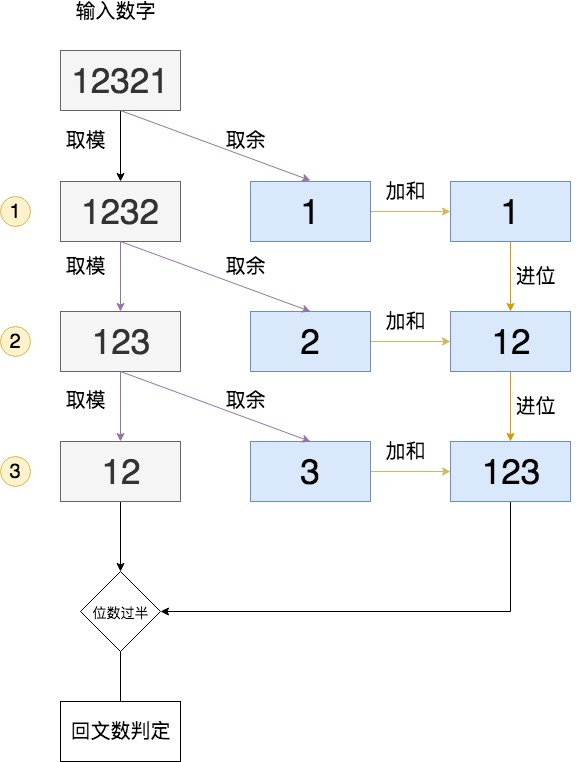# 回文数判断 Palindrome Number

## 题目

Determine whether an integer is a palindrome. An integer is a palindrome when it reads the same backward as forward.

Example 1:

``````Input: 121
Output: true
``````

Example 2:

``````Input: -121
Output: false
Explanation: From left to right, it reads -121. From right to left, it becomes 121-. Therefore it is not a palindrome.
``````

Example 3:

``````Input: 10
Output: false
Explanation: Reads 01 from right to left. Therefore it is not a palindrome.
``````

Coud you solve it without converting the integer to a string?

## 思路&题解1. 负数排除
2. 个位数是0的多位数排除
3. 0本身是回文数``````public class Solution {
public boolean isPalindrome(int x) {
// 易错点：特殊值需要提前排除
if(x < 0 || (x%10 == 0 && x != 0)){
return false;
}

int result = 0;
// 易错点：判断位数达到一半
while(x > result) {
result = result*10 + x%10;
x /= 10;
}
// 易错点：对半判断
return result == x || result/10 == x;
}
}
``````

## 知识点分析

• 回文数的规律&特殊值 负数排除是比较直观的，但是10的倍数也需要排除。除了0之外，由于整数高位不能是0，因此个位数是0的整数翻转后不是回文数。

• 如何判断位数的一半

// TODO 需要考虑溢出问题

``````int num = 10000;
int count = 1;
while ((num / (int)Math.pow(10, count)) > 0) {
count++;
}
System.out.println(num + "=>" + count);
``````